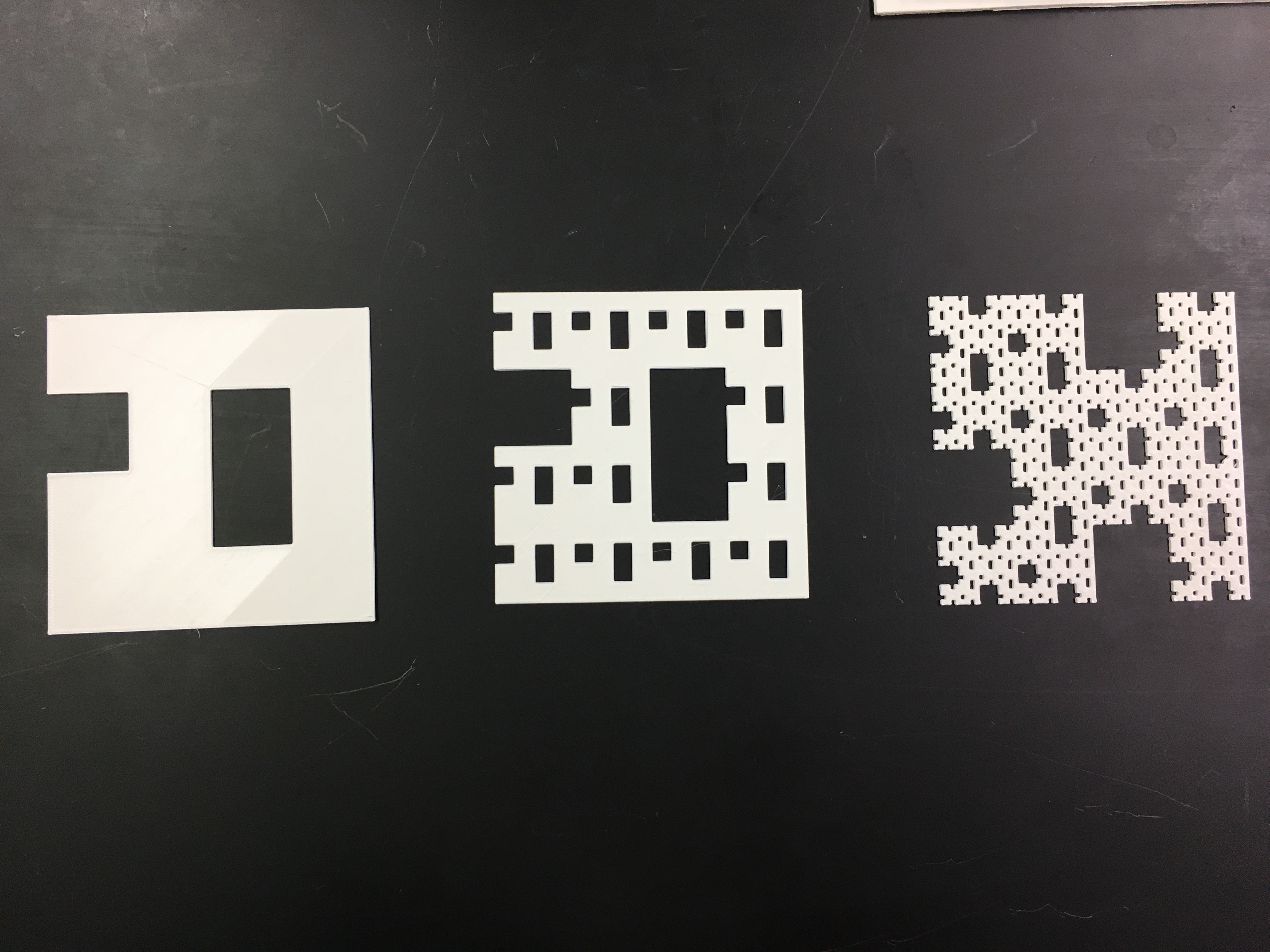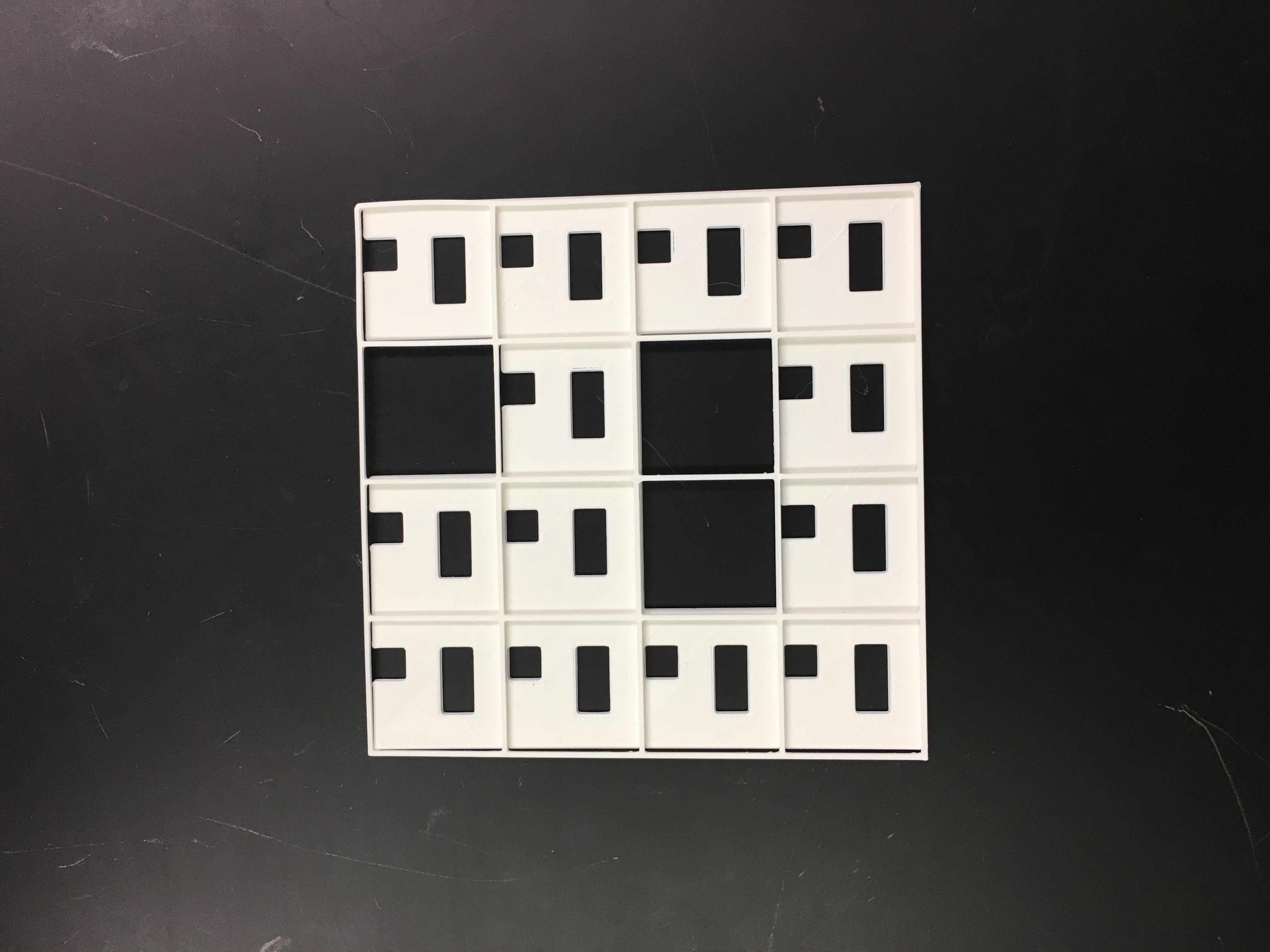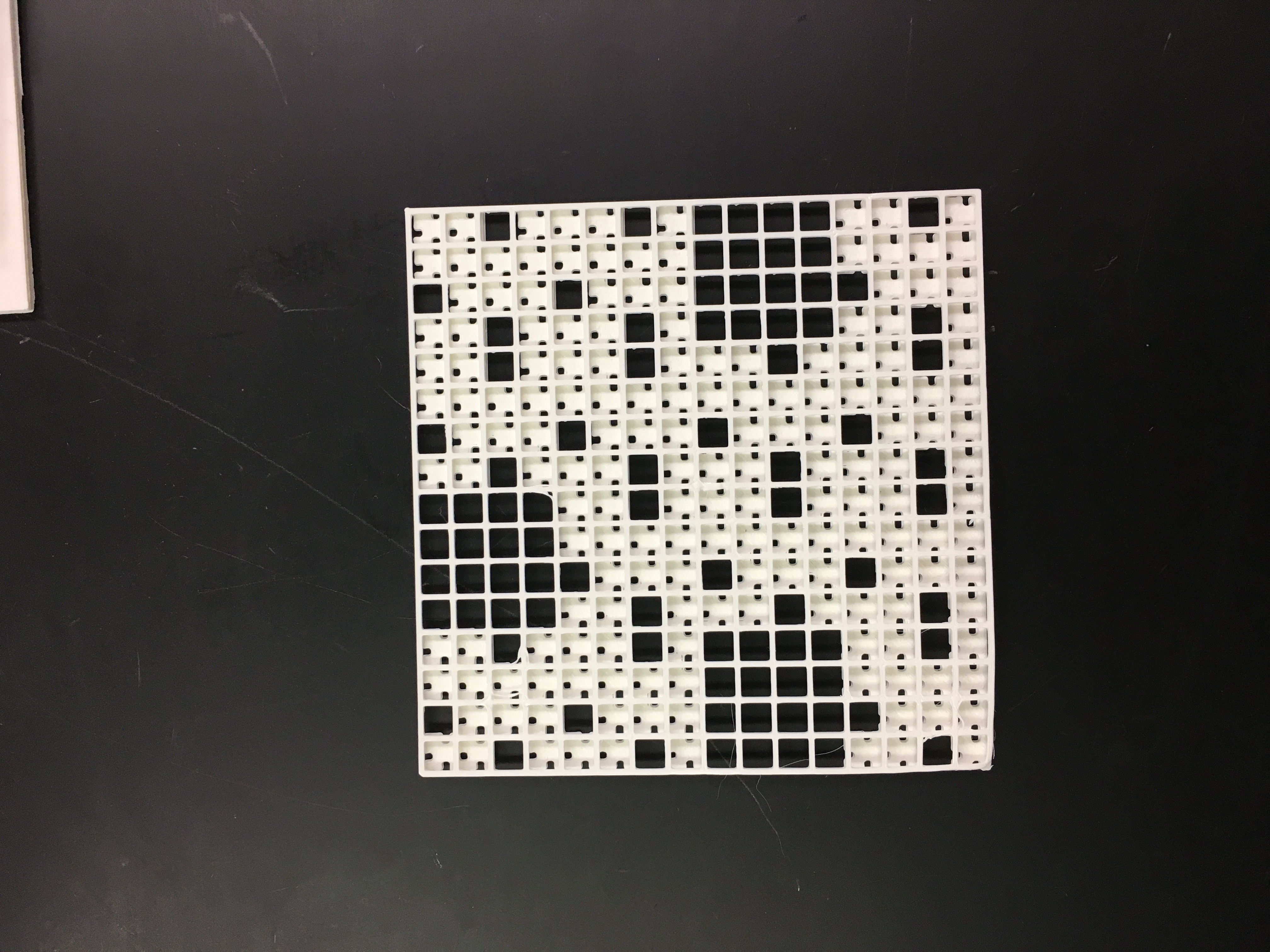# Computer RAM Carpet Fractal

### Definition

In my fractal, I removed 3 coordinates, [2,1], [0,2], and [2,2].

### Surface Area Calculations

#### Level 1

16 – (13)

Before I removed squares in my fractal, there were 16 squares in total. For my level 1, I removed 3 squares, leaving 13 in the fractal. The area for level one is 13.

#### Level 2

16 – (13) – ((13 * 3) (1/16))

For level 2, I multiplied the 13 remaining squares I had by the 3 squares I removed. Then, I multiplied that number by 1/16 because that is the area of each remaining square. The equation above equals 2.4375 but then we must subtract that from the area of level 1, 13-2.4375 = 11.5625 ft^2.

#### Level 3

16 – (13) – ((13 * 3) (1/16)) – (13(13 * 3) (1/256))

For level 3, I multiplied 13 by 3 again but this time I multiplied that number by 13 because for level 3, 13 times more squares were added. Then, I multiplied that amount by 1/256, which is 1/16^2, because that is the area of each remaining square. The equation above equals 1.9805 but we must subtract the area of level 2 from that, 11.5625 – 1.9805 = 9.5820 ft^2Surface Area of “Infinite” Levels

16 – (3(1)) – (3(1/16) (12)) – (3(1/16²) (13)²) – (3(1/16³) (13)³) – (3(1/16^4) (13)^4)

16 [ (3) + 3(13/16) + 3(13/16)² + 3(13/16)³ + 3(13/16)^4 ]

16 – (3 x 1/1-13/16)

I started out my sum of an infinite geometric series with the number of squares I started with, 16. Then, I plugged my values into A (3) x 1/1-r (13/16). In order to solve what numbers I would need to plug in for A and r, I set out my equation for the surface area up to the fourth power. After level 1, each level was the same except its power was increased by 1.

Dimension

(1/4)^D = 1/13

4^D = 13

log4 13 = D

D ≈ 1.8502

As the fractal carpet grows, it is scaled down by 1/4 to create an identical square. After locating a square that looked identical to the level 1 fractal, I can clearly see that the area is 13. As the video presented to us, my formula will be (1/4)^D = 1/13. Then, I plugged in my values to the formula A^D = B, which translates to logA B = D. My formula was then 4^D = 13 and then log4 13 = D. When I plugged that equation into my calculator, I received the number 1.8502.